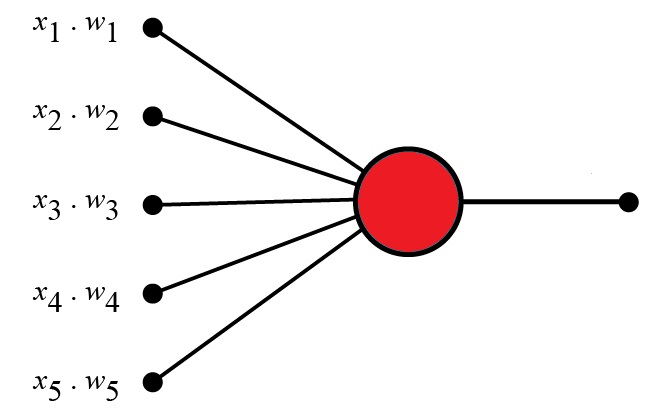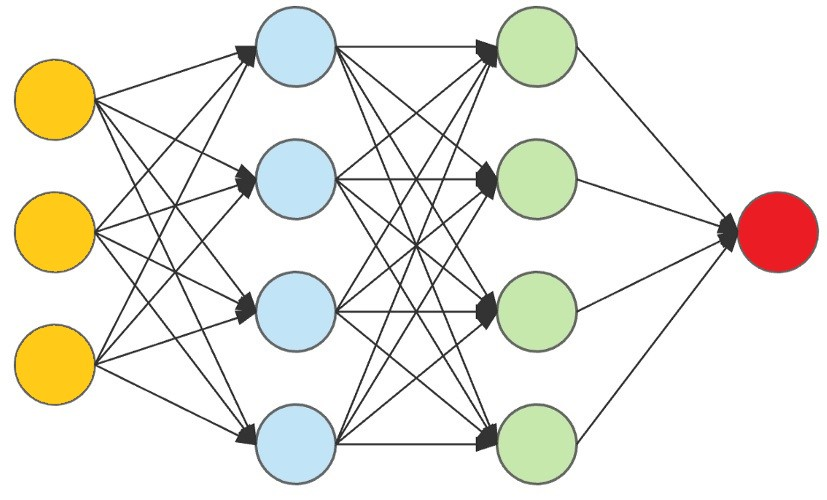# Perceptrons

A Perceptron is an Artificial Neuron

It is the simplest possible Neural Network

Neural Networks are the building blocks of Machine Learning.

## Frank Rosenblatt

Frank Rosenblatt (1928 – 1971) was an American psychologist notable in the field of Artificial Intelligence.

In 1957 he started something really big. He "invented" a Perceptron program, on an IBM 704 computer at Cornell Aeronautical Laboratory.

Scientists had discovered that brain cells (Neurons) receive input from our senses by electrical signals.

The Neurons, then again, use electrical signals to store information, and to make decisions based on previous input.

Frank had the idea that Perceptrons could simulate brain principles, with the ability to learn and make decisions.

## The Perceptron

The original Perceptron was designed to take a number of binary inputs, and produce one binary output (0 or 1).

The idea was to use different weights to represent the importance of each input, and that the sum of the values should be greater than a threshold value before making a decision like yes or no (true or false) (0 or 1).## Perceptron Example

Imagine a perceptron (in your brain).

The perceptron tries to decide if you should go to a concert.

Is the artist good? Is the weather good?

What weights should these facts have?

CriteriaInputWeight
Artists is Goodx1 = 0 or 1w1 = 0.7
Weather is Goodx2 = 0 or 1w2 = 0.6
Friend will Comex3 = 0 or 1w3 = 0.5
Food is Servedx4 = 0 or 1w4 = 0.3
Alcohol is Servedx5 = 0 or 1w5 = 0.4

## The Perceptron Algorithm

Frank Rosenblatt suggested this algorithm:

1. Set a threshold value
2. Multiply all inputs with its weights
3. Sum all the results
4. Activate the output

1. Set a threshold value:

• Threshold = 1.5

2. Multiply all inputs with its weights:

• x1 * w1 = 1 * 0.7 = 0.7
• x2 * w2 = 0 * 0.6 = 0
• x3 * w3 = 1 * 0.5 = 0.5
• x4 * w4 = 0 * 0.3 = 0
• x5 * w5 = 1 * 0.4 = 0.4

3. Sum all the results:

• 0.7 + 0 + 0.5 + 0 + 0.4 = 1.6 (The Weighted Sum)

4. Activate the Output:

• Return true if the sum > 1.5 ("Yes I will go to the Concert")

## Note

If the weather weight is 0.6 for you, it might be different for someone else. A higher weight means that the weather is more important to them.

If the threshold value is 1.5 for you, it might be different for someone else. A lower threshold means they are more wanting to go to any concert.

### Example

const threshold = 1.5;
const inputs = [1, 0, 1, 0, 1];
const weights = [0.7, 0.6, 0.5, 0.3, 0.4];

let sum = 0;
for (let i = 0; i < inputs.length; i++) {
sum += inputs[i] * weights[i];
}

const activate = (sum > 1.5);

Try it Yourself »

## Perceptron Terminology

• Perceptron Inputs (nodes)
• Node values (1, 0, 1, 0, 1)
• Node Weights (0.7, 0.6, 0.5, 0.3, 0.4)
• Activation Function (sum > treshold)

## Nodes (Perceptron Inputs)

Perceptron inputs are called nodes.

The nodes have both a value and a weight.

## Node Values (Input Values)

Each input node has a binary value of 1 or 0.

This can be interpreted as true or false / yes or no.

In the example above, the node values are: 1, 0, 1, 0, 1

## Node Weights

Weights shows the strength of each node.

In the example above, the node weights are: 0.7, 0.6, 0.5, 0.3, 0.4

## The Activation Function

The activation function maps the the weighted sum into a binary value of 1 or 0.

This can be interpreted as true or false / yes or no.

In the example above, the activation function is simple: (sum > 1.5)

## Note

It is obvious that a decision is NOT made by one neuron alone.

Many other neurons must provide input:

• Is the artist good
• Is the weather good
• ...

Multi-Layer Perceptrons can be used for very sophisticated decision making.

## Neural Networks

The Perceptron defines the first step into Neural Networks:W3Schools is optimized for learning and training. Examples might be simplified to improve reading and learning. Tutorials, references, and examples are constantly reviewed to avoid errors, but we cannot warrant full correctness of all content. While using W3Schools, you agree to have read and accepted our terms of use, cookie and privacy policy.